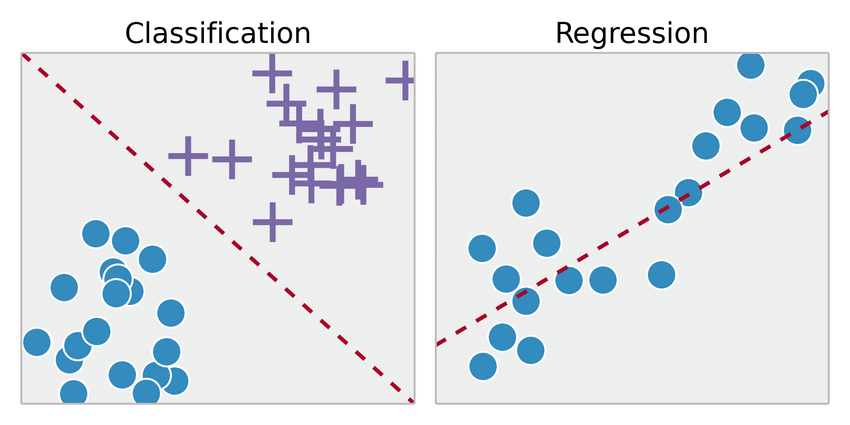## Difference between classification and regression

Classification and Regression happen to be two key problems in prediction that are handled by data mining and ML.

### Classification

It is the method by which a model or function is discovered that helps to segregate the data into various categorical groups, i.e. discrete values. In classification, the data is labeled according to certain input criteria under different labels and then the labels for the data are expected. Classification is a tool that can be used for categorizing a given set of data into groups on both structured and unstructured data. The method starts with the projection of the class of given data points. Classes are also regarded as targets, labels, or categories. Following are some of the classification algorithms:

Check out the Data Science Masters Course @ FingerTips

### Logistic Regression

The primary idea of logistic regression is to find relationships between features an the probability of particular outcome.

Decision Tree: Decision tree is a tree-structured classifier, where internal nodes represent the features of a dataset, branches represent the decision rules and every leaf node represent the outcome.

Support Vector Machines (SVM): The SVM algorithm's goal is to build the best line or decision boundary that can segregate n-dimensional space into groups such that in the future we can conveniently place the new data point in the right group.

KNN: The KNN algorithm assumes that identical items exist in close proximity. In other words, similar things are close to each other. The principle of similarity (sometimes called distance, proximity, or closeness) is captured by KNN with some calculation, measuring the distance on a graph between points.

Naïve Bayes Classifiers

Naive Bayes classifiers are a set of Bayes' Theorem-based classification algorithms. It is not a single algorithm but a family of algorithms where a general concept is shared by all of them, i.e. each pair of features being classified is independent of each other.

Regression:

For predicting a continuous value, regression models are used. One of the typical examples of regression is the estimation of house prices given house characteristics such as size, location, price, etc.  Using training data, a single output value is generated in regression. This value is a probabilistic interpretation that is computed after taking into account the frequency of the correlation between the input variables. Following are some Regression Algorithms:

Simple Linear regression: One of the variables is predictor or independent variable and the other is response or dependent variable. It attempts to find statistical relationship but not deterministic relationship.

Multiple Linear Regression: In order to estimate the relationship between two or more independent variables and one dependent variable, multiple linear regression is used.

Polynomial Regression:  Assuming we know that our data is correlated, but the relationship doesn’t look linear? So depending on what the data looks like, we can do a polynomial regression on the data to fit a polynomial equation to it.

##### Tags: